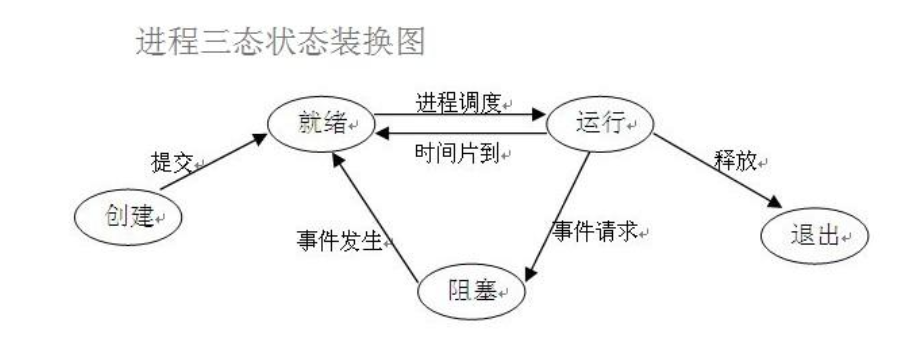## 进程的调度算法

• 先来先服务(FCSC)：对长作业有利，对短作业无利
• 短作业优先调度算法(SPN)：对短作业有利，对长作业无利。
• 时间片轮转法+多级反馈

时间片轮转：将CPU的执行时间进行划分，比如每个进程执行x毫秒或者执行中遇到IO，就切换到下一个进程。

(1) 应设置多个就绪队列，并为各个队列赋予不同的优先级。第一个队列的优先级最高，第二个队列次之，其余各队列的优先权逐个降低。该算法赋予各个队列中进程执行时间片的大小也各不相同，在优先权愈高的队列中，为每个进程所规定的执行时间片就愈小。
(2) 当一个新进程进入内存后，首先将它放入第一队列的末尾，按FCFS原则排队等待调度。当轮到该进程执行时，如它能在该时间片内完成，便可准备撤离系统；如果它在一个时间片结束时尚未完成，调度程序便将该进程转入第二队列的末尾，再同样地按FCFS原则等待调度执行；如果它在第二队列中运行一个时间片后仍未完成，再依次将它放入第三队列，……，如此下去。

(3) 仅当第一队列空闲时，调度程序才调度第二队列中的进程运行；仅当第1～(i-1)队列均空时，才会调度第i队列中的进程运行。若cpu在执行优先级低队列时,有程序进入高优先级队列，则会立刻将此进程放入队列末尾，把CPU分配给新到高优先级进程。

## 进程三状态切换## 进程的创建

winows和linux中进程的区别：

• windows中创建进程时发生什么？

1. 系统会将原来的代码复制
2. 运行一遍代码以产生命名空间
3. 运行创建进程时指定的函数
• linux中创建进程会发生什么？

1. 调用系统的fork语句
2. fork将当前代码的运行空间复制一份，包括目前运行到的状态
3. 原进程中的fork返回创建出来的进程号，新创建出来的进程的fork返回0

• windows中需要进行`__main__`的判断,防止循环创建
• windows中会运行完代码后才调用进程要调用的函数

### python中创建进程

``````# 最常使用的方法
from multiprocessing import Process
import time

def task(name):
print('我被创建了')

if __name__ == '__main__':
# 1 创建一个对象
p = Process(target=task, args=('shuta',))
# 2 开启进程
p.start()  # 告诉操作系统帮你创建一个进程  异步
print('hello')

# 第二种方式 类的继承
from multiprocessing import Process
import time

class MyProcess(Process):
def run(self):
print('我被创建了')

if __name__ == '__main__':
p = MyProcess()
p.start()
print('hello')
# 使用is_alive可以判断进程是否存活
p.is_alive() ``````
``````# 查看进程号
import os

# 当前进程号
os.getpid()
# 父进程号
os.getppid()``````

### 使用join等待进程的执行

``````from multiprocessing import Process
import time

def task(name, n):
print('进程被创建了')
time.sleep(1)

if __name__ == '__main__':
p = Process(target=task, args=('jason', 1))
start_time = time.time()
p.start()
p.join() # p进程执行结束后，才会执行后边的语句
print('hello', time.time() - start_time)``````

### 使用守护进程

``````from multiprocessing import Process
import time

def task():
print('进程被创建')
time.sleep(3)
print('进程结束')

if __name__ == '__main__':
p = Process(target=task,)
# 在进程启动前，设置为守护进程
p.daemon = True
p.start()
print('主进程结束')``````

### 进程中使用互斥锁

``````# 多线程模拟买票的案例，票的信息存储在文件中
from multiprocessing import Process, Lock
import json
import time
import random

# 查票
def search(i):
# 文件操作读取票数
with open('data','r',encoding='utf8') as f:
dic = json.load(f)
print('用户%s查询余票：%s'%(i, dic.get('ticket_num')))
# 字典取值不要用[]的形式 推荐使用get  这样不会报错！！！

# 买票  1.先查 2.再买
def buy(i):
# 先查票
with open('data','r',encoding='utf8') as f:
dic = json.load(f)
# 模拟网络延迟
time.sleep(random.randint(1,3))
# 判断当前是否有票
if dic.get('ticket_num') > 0:
# 修改数据库 买票
dic['ticket_num'] -= 1
# 写入数据库
with open('data','w',encoding='utf8') as f:
json.dump(dic,f)
print('用户%s买票成功'%i)
else:
print('用户%s买票失败'%i)

# 整合上面两个函数
def run(i, mutex):
search(i)
# 给买票环节加锁处理
# 抢锁
mutex.acquire()

buy(i)
# 释放锁
mutex.release()

if __name__ == '__main__':
# 在主进程中生成一把锁 让所有的子进程抢 谁先抢到谁先买票
mutex = Lock()
for i in range(1,11):
p = Process(target=run, args=(i, mutex))
p.start()``````

### 进程间通讯

#### queue的使用

``````form multiprocess import  Queue

# 创建队列，传入队列的大小
q = Queue(5)

# 往队列中存数据
q.put(111)
q.put(222)

# 在队列中取数据
v1 = q.get()
v2 = q.get()

v = q.get_nowait()  # 没有数据直接报错queue.Empty
v = q.get(timeout=3)  # 没有数据之后原地等待三秒之后再报错

# 后进先出队列
q = queue.LifoQueue(3)

# 优先级排序队列
q = queue.PriorityQueue(4)
q.put((10, '111'))
print(q.get()) ``````

#### 进程间通讯（IPC）

``````# 借助与队列的通讯（生产者与消费者模型）
from multiprocessing import Queue, Process

def producer(q):
q.put('生产的数据')

def consumer(q):
print(q.get())

if __name__ == '__main__':
q = Queue()
p = Process(target=producer,args=(q,))
p1 = Process(target=consumer,args=(q,))
p.start()
p1.start()``````

### 进程池

1. 频繁的创建销毁进程会降低计算机的执行效率
2. 提前将进程创建好可以提高请求到来时的处理速度
3. 防止同时的请求过多，消耗系统过多的资源，降低的系统的请求处理速度

``````from concurrent.futures import ThreadPoolExecutor
import time
import os

# 1. 设置好进程池的大小，并生成进程池
# 括号内可以传数字 不传的话默认会开设当前计算机cpu个数五倍的线程
pool = ThreadPoolExecutor(5)  # 池子里面固定只有五个线程

def task(n):
print(n,os.getpid())
time.sleep(2)
return n**n

def call_back(n):
print('call_back>>>:',n.result())

'''

同步:提交任务之后原地等待任务的返回结果 期间不做任何事
异步:提交任务之后不等待任务的返回结果 执行继续往下执行
返回结果如何获取？？？
异步提交任务的返回结果 应该通过回调机制来获取
一旦该任务有结果立刻触发回调
'''
# 使用同步的方式提交
if __name__ == '__main__':
# 朝池中提交任务,传入需要的参数
pool.submit(task, 1)
print('主')
t_list = []
# 朝池子中提交20个任务
for i in range(20):
# 任务完成后，调用call_back方法
res = pool.submit(task, i).add_done_callback(call_back)

``````

``````if __name__ == '__main__':
pool.submit(task, 1)  # 朝池子中提交任务  异步提交
print('主')
t_list = []
for i in range(20):  # 朝池子中提交20个任务
res = pool.submit(task, i)
print(res.result())  # result方法   同步提交
t_list.append(res)
# 等待池中所有的任务执行完毕之后，关闭线程池，再继续执行后续的代码
pool.shutdown()
for t in t_list:
print('>>>:',t.result())  ``````

queue是如何实现的